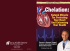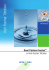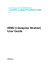# Complex forming reactions and complexometry Complex forming

## Transcription

Complex forming reactions and complexometry Complex forming
```2011.01.10.
Complex forming reactions
and complexometry
Gábor Galbács
Complex forming reactions
Mixing of salt solutions usually does not result in any observable
changes. The properties of the species originally present persist
and no new properties can be detected (e.g. no precipitate, no
color change, etc.). Examples:
NaCl + KNO3
or
ZnSO4 + MgCl2
But it is not always the case… see, for instance:
FeCl3
+
yellow
CoCl2
pink
KF
→
colorless
+
KSCN
colorless
?
colorless
→
?
blue
plus, metal ions are also hidden from their usual reactions…
1
2011.01.10.
Complex forming reactions
In these cases, the reaction between the ions resulted in the
formation of complex ions (compounds):
Fe3+ + 6 F- = [FeF6]3Co2+ + SCN- = [Co(SCN)]+
Complex compounds contain a central metal ion surrounded by
other ion(s) or molecule(s) called ligands. Ligands can be ions
or neutral molecules (e.g. ammonia, water).
The driving force behind the formation of complex ions is that
certain atoms in the ligands donate one of their free electron
pairs to the central metal ion (donor atoms), to fill its valence
shell. This is essentially an acid-base reaction according to Lewis.
This bond type is called coordinative bond.
Complex forming reactions
The number of electron pairs a metal ion is capable of receiving
depends on it radius and electron configuration (e.g. the M shell
of Si4+ can accept a total of 18 electrons) and also the structure
of the ligand. This number is called the coordination number.
If there is only one donor atom in the ligand, it is called
monodentate, if two then it is bidentate, etc. Examples:
coordination number: 6
monodentate ligands
coordination number: 4
bidentate ligands
2
2011.01.10.
Complex forming reactions
If there is more than one type of ligand present in a complex, it
is called a mixed complex. Example:
[Fe(H2O)4(SCN)2]+
In some complexes, there may be even more than one central
metal ion; these are multi center complexes. Examples:
Mn2(CO)10
Fe2(CO)9
Nomenclature of complex compounds
The formula of a complex ion is put in square brackets, and it is
placed at the front if its charge is positive, or at the back if
negative. Examples:
[C (NH3)6]Cl3
[Co(NH
K3[Fe(CN)
[F (CN)6]
The name of complex cations starts with the greek form of the
coordination number (mono-, di-, tri-, etc.), and then comes the
name of the ligand (an –o tag is also appended to it if the ligand
itself has charge), plus the name of the metal ion with its valence
state indicated. Examples:
[Ag(NH3)2]+
[Ni(H2O)6]2+
[CuCl4]2-
diammine-silver (I) ion
hexaaqua-nickel (II) ion
tetrachloro-copper (II) ion
3
2011.01.10.
Nomenclature of complex compounds
Naming of complex anions is similar, but with an -ate ending
added to the name of the metal ion. Examples:
[BiI4][Al(OH)4][FeF6]-
tetraiodo-bismutate (III) ion
tetrahydroxo-aluminate (III) ion
hexafluoro-ferrate (III) ion
In the case of a neutral complex:
[HgCl2]
[Fe(SCN)3]
dichloro-mercury (II)
tri-thiocyanato-iron (III)
And finally, if they are written up with the counterions:
[Cu(NH3)4]SO4
Na3[Ag(S2O3)2]
[tetraammine-copper(II)]-sulphate
sodium-[dithiocyanato-argentate]
Structure of complex compounds
Coordinated ligands always try to obtain positions around the
central metal ion so that the compound becomes energetically
the most stable – that is the structure tends to be symmetrical.
Examples:
p
coordination number: 2
structure is linear
coordination number: 4
structure is tetrahedral
coordination number: 6
structure is octahedral
coordination number: 5
structure is trigonal-bipiramidal
4
2011.01.10.
Structure of complex compounds
Complex formation equilibria
Complex reactions always commence in a stepwise fashion. Each
equilibrium is characterized by a stepwise formation constant
(the example below is given for e.g. a complex with a
coordination number of 4 and monodentate ligands):
M + L ↔ ML
K1 =
[ML]
[M] ⋅ [L]
ML + L ↔ ML 2
K2 =
[ML 2 ]
[ML] ⋅ [L]
ML 2 + L ↔ ML 3
K3 =
[ML 3 ]
[ML 2 ] ⋅ [L]
ML 3 + L ↔ ML 4
K4 =
[ML 4 ]
[ML 3 ] ⋅ [L]
where usually K1 ≥ K2 ≥ K3 ≥ K4.
5
2011.01.10.
Complex formation equilibria
The overall formation constant (K, β) for the last complex in
the chain is the product of the formation constants.
β = K1 · K2 · K3 · K4
M + n L ↔ MLn
β =
[MLn ]
[M] ⋅ [L]n
Because of the parallel equilibria, there is a number of species
present in a solution containing complex compounds (e.g. M, L,
ML ML1, ML2, ML3, ML4).
ML,
) The concentration of these species is
calculable, even if in a complicated manner, using the formation
constants.
One can also see that full complexation of a metal ion is only
possible using a large excess of the ligand.
Conditional formation constant
The formula for the overall formation constant suggest that the
complex formation is only governed by the concentration of the
ligand, and the free metal ion. For a hexadentate ligand (EDTA,
Y4-),
) as an example,
example it is:
In reality, the formation equlibrium is also affected by any side
reactions that influence [Y4-] or [Mn+]. These include:
•p
pH ((since p
protons compete
p
with metal ions for the ligand)
g
)
• other ligands (as they compete with ligand for the metal ion)
These effects can be taken into account by introducing the so
called virtual (conditional) formation constant. As an
illustration, we will discuss the effect of pH in the followings.
6
2011.01.10.
Conditional formation constant
The ligand not complexed is present in various protonated forms.
If we denote the total dissolved ligand concentration by Y’, then
we can write
[Y’] = [Y4-] + [HY3-] + [H2Y2-] + [H3Y-] + [H4Y]
and we can define a proportionality factor:
αH = [Y’]/[Y4-]
The value for this factor is, of course, calculable based on the
p
dissociation equilibria
q
for H4Y. If we do the math,, we
stepwise
obtain:
αH = 1 + K1⋅[H+] + K1⋅K2⋅[H+]2 + K1⋅K2⋅K3⋅[H+]3 + K1⋅K2⋅K3⋅K3⋅[H+]4
Conditional formation constant
Knowing the constants from K1 to K4 ,
the function can be plotted graphically
against the pH.
Using this newly introduced factor we
can write:
This means that we can calculate a
conditional formation constant,
which refers to the actually present
total ligand concentration, if we know
αH (as a function of pH).
7
2011.01.10.
Chelate complex formation
Certain complex compounds that are formed with multidentate
ligands in a way that five- or six-membered ringlike structures
are created around the metal ion, are very stable (see the
example below).
below) These rings are called chelate rings and the
complexes chelates.
These complexes have great importance in complexometry.
Chelate forming ligands (complexons)
nitrilo-triacetic acid (NTA)
ethylene-diammine-tetraacetic acid (EDTA)
1,2-diamino-cyclohexane-tetraacetic acid (DCTA)
Comment: EDTA has four acetate groups and two amine nitrogens (all only
accessible when deprotonated), which means a total of 6 binding sites (dents)
Thus, EDTA is often abbreviated as Y4-.
8
2011.01.10.
Structure of an EDTA chelate complex
Complexometry
Complexometry is a titrimetric method that is primarily used for
the determination of metal ions, with the aid of complex
formation (mainly chelate) reactions.
The following conditions need to be met for the successful use of
a complex reaction in titrimetry:
• the complex needs to be very stable, with a high formation
constant so the reaction is stochiometric (chelates)
• the solution needs to be buffered
• the complex needs to be formed in 1:1 ratio
(for a sharp end-point)
There are several useful complexons, but by far the most useful
is ethylene-diammine tetraacetic acid or EDTA.
9
2011.01.10.
Complexometry
EDTA has many features useful in complexometry:
• it is a versatile titrant, as it forms highly stable 1:1 ratio
chelate complexes with a number of metals
• its own color does not interfere with indicators, as
EDTA solution is colorless, and so are most of its complexes
• EDTA and also its metal complexes are water soluble
• EDTA solutions are stable (in plastic containers)
• it is relatively harmless (non-toxic)
Versatility of EDTA complexometry
10
2011.01.10.
Complexometric titration curves
Titration curves usually plot the pM against the added titrant
(EDTA) volume. Note, that an increasing pM means strongly
decreasing [M]. Points of the titration curve can be calculated
using the conditional formation constant K’ (at fixed pH). If K’ is
large, we can assume a complete reaction at each point.
Example:
50 mL 0.05 M Ca2+
0.05 M EDTA (Y) titrant
K’ = 1.3 × 1010
M + Y ↔ MY
Before the equivalence point:
pM is controlled by the excess, unreacted metal ion
At the equivalence point:
M is only present due to the slight dissociation of MY
After the equivalence point:
pM is controlled by the excess EDTA
Complexometric titration curves
REGION 1 - BEFORE THE EQUIVALENCE POINT
pM= -lg [M] = -lg (0.05) = 1.301
we can neglect the dissociation of MY
pM= -lg [Mremaining]
cM,
remaining=
((cM, total·VM) - (cEDTA·VEDTA, added)) / Vtotal
pM = -lg (0.0333) = 1.477
the approach is the same as above
pM = - lg (0.0166 M) = 1.778
11
2011.01.10.
Complexometric titration curves
REGION 2: AT THE EQUIVALENCE POINT
50 mL titrant was added (100%):
[MY ]
= K′
[M][Y ]
We can calculate pM like we dissolved MY
First, we calculate the total [MY] and then pM will be
determined by the dissociation.
[MY]= (cM, total·VM) / Vtotal = 0.025 M
0.025− x
= 1.3 ⋅ 1010 ⇒ x = 1.38⋅ 10−6M
2
x
pM= -lg (1.38 · 10-6) = 5.85
Complexometric titration curves
REGION 3: AFTER THE EQUIVALENCE POINT
We use the equilibrium formula again,
again
but with the actual concentrations:
[MY]
= K′
[M][Y]
[MY]= (cM, total·VM) / Vtotal = 0.020 M
[EDTA] = (cEDTA·VEDTA, excess) / Vtotal = 0.010 M
0.02
= 1.3 ⋅ 1010 ⇒ [M] = 1.53 ⋅ 10 −10 M
[M] ⋅ 0.01
pM= -lg (1.53 · 10-10) = 9.81
12
2011.01.10.
Complexometric titration curves
Electrode signal proportiona
al to [Ca2+]
Effect of K and pH on titration curves
the larger is K, the larger is the jump
on the titration curve around the EP
Simulation from Skoog CD-ROM (Part III, Module 19)
at higher pH values, EDTA titration curves
usually exhibit more distinct EP (less
competition from protons), but beware of
hydroxide precipitatiton…
13
2011.01.10.
Complexometry – auxiliary agents
Some metal ions need to be titrated at such alkaline pHs, which
normally cause their hydroxide precipitate to form. To prevent
this one can add complexing agents to the sample which bring
this,
the metal ion into a weak complex. This weak complex will
prevent the metal ions from forming hydroxides, but are weak
enough to give up the metal ions upon the addition of EDTA.
Examples to such agents:
• ammonia
• tartarate
• citrate
14
2011.01.10.
End point indication in complexometry
EP indication is done by weak complexing agents that change
their color upon complexation (metal ion indicators). Here is
what happens during titration:
indicator some metal ions will react with
it, so color will be that of the indicator-metal complex
(MIn)
• the added EDTA will react first with free metal ions
• near, but before the end-point MIn gives up its metal ion
to EDTA, and the more stable EDTA-metal complex forms
• at the end-point, all MIn is broken up, so the indicator will
show its free color
Of course, the indicator-EDTA push-pull reaction needs to be
fast, so the end-point will not be overrun. It also follows that
there is no indicator error in complexometry, thus we can use a
larger amount of indicator if needed.
End point indication in complexometry
15
2011.01.10.
Masking can be used to protect some component of the sample
to react with EDTA. Masking agents are strong complexants.
Examples:
• F- masks Al3+, Fe3+, Ti4+, Be2+
• CN- masks Cd2+, Zn2+, Co2+, Ag+, etc.
• triethanolamine masks Al3+, Fe3+, Mn2+
If the masked component later also needs to be titrated by EDTA,
release the metal ion from a masking agent. Examples:
Preparation of the titrant solution
The titrant is usually 0.02-0.05 M EDTA solution in
complexometry, prepared by the dissolution of EDTA or its Na
salt.
The titrant needs to be standardized due to its high affinity
towards metal ions. The standardization can be done by using
e.g. a standard Zn(II) solution, either prepared from the pure
metal or from its salt.
Due to metallic impurities in glass containers, plastic containers
are recommended for storage.
storage
16
2011.01.10.
Applications of complexometry
Complexometric applications always need a careful setting of the
pH, for which one uses buffer solutions. Care must be exercised
when choosing the buffer, as these contain weak bases and/or
acids that may also have complexing properties (e.g. ammonia,
citratic acid,
d acetic acid,
d etc.))
Direct titration of metal ions
A number of metal ions can be titrated directly. See the former
tables for this.
Indirect titration
Anions
o
that
a p
precipitate
p a
with certain
a metal
a ions
o
can
a b
be a
analyzed
ay d
with EDTA indirectly. For example, SO42- can be first precipitated
from the sample solution using excess Ba2+ as BaSO4. Then the
precipitate is washed and separated from the sample solution and
boiled with excess EDTA at pH= 10 to bring all Ba2+ back into
solution as Ba(EDTA)2-. The excess of EDTA can be then titrated
back using e.g. Mg2+.
Applications of complexometry
Water hardness determination
Hardness is the total alkaline earth ion concentration in water
(these ions form a precipitate with soap, hence the „hard water”
expression).
p
) These are mainly
y Ca2+ and Mg
g2+, so by
y titrating
g
these two ions with EDTA, one can assess the hardness of a
water sample.
Sample preparation: masking of minor metal ions in the sample
that may also react with EDTA has to be done (ascorbic acid for
Fe3+ reduction, CN- for Fe2+, Cu+, etc.)
If the titration is done at pH = 10 in ammonia buffer, the result
gives the total [Ca2+]+[Mg2+]. Indicator: Eriochrome Black T
Ca2+ can be titrated separately at pH= 13 without ammonia. At
this pH, Mg(OH)2 precipitates and so Mg2+ is inaccessible to
EDTA. Indicator: Murexide
17
2011.01.10.
Summary of EDTA complexometry
Main use:
titrimetric determination of metal ions
Main appeal: 1) very versatile titrant
2) easily calculable,
calculable uniform stoichiometry
3) easy EP indication, largely free from error
Titrant:
0.02-0.05 M solution, needs to be standardized
using metal ion solutions
Conditions:
sample has to be buffered (usually to an alkaline pH)
Indicators:
weak complexing agents (Murexide, Xylenol orange, etc.)
Applications: e.g. direct titration of metal ions, water hardness
determination, indirect sulphate determination, etc.
18
```

### Recycling Bloopers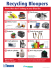### Experiment 5. Volumetric Determination of Calcium in TUMS® via EDTA... Techniques Weighing TCD-4,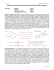### TEA STAINED STAINLESS STEEL?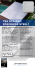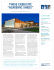### PDF(English) - Ccis.nic.in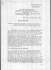### 10` x 8` Del Mar Metal Storage Shed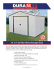### TM - Bradley`s Auction Service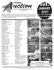### BETTY VANAMBURG - Easter and Associates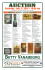### Lo Down Magazine - Metal Spirit Resurrection### Know More..... - Wood Seasoning Machinery Suppliers | Air Curtains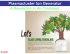### steel construction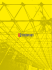### DEIONIZED WATER Experiment. EDTA Titration of Zinc in an unknown sample Objective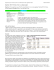### Purification of natural zeolite-clinoptilolite for medical application-Extraction of lead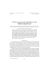### Detoxamin - Chelation Research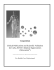### Chelation - Natura Naturans